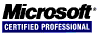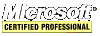# .NET C# Guide

## Program Control Statements

Program control statemens are statements that control a program's flow of execution.

### if else

Selectively execute part of a program through the use of the conditional statement `if` and `else`.

``````int i = 3;

if(3 == i) i++; // this statement consumes a single line which is OK to do

if(4 == i)
i++; // i equals 4 so this line will execute
else
i--; // this line will no execute

if(5 == i)
{
i++; // inside a code block (see page 1 of this guide)
}
else
i--;
``````

`if` statements can be within `if` statements. This is known as nesting.

### for

The `for` loop has this syntax:

`for(initialization; condition; iteration) statement;`

``````double d = 0.0;

for(int i = 0; i < 10; i++) // i will go from 0 to 9 and then stops when it hits 10
d += i * 1.25; // this executes 10 times

for(int i = 1; i <= 10; i++) // i will go from 1 to 10 and then stops when it hits 11
{
d += i * 1.25; // this executes 10 times
}
``````

`for` loops can be nested.

### while

The `while` loop has this syntax:

`while(condition) statement;`

``````int x = 0;
char c = 'z';

while(c >= 'a')
{
x += c--;
}
``````

### do while

The `do while` loop checks its condition at the end after it has executed its block. It has this syntax:

`do { statement; } while(condition);`

``````int x = 0;
char c = 'z';

do
{
x += c--;
}
while(c >= 'a');
``````

### break

It is possible to immediate exit a loop using a `break` statement.

``````double d = 0.0;

for(int i = 0; i < 10; i++)
{
d += i * 1.25;
if(d >= 100.0)
break; // exit the loop
}
``````

### continue

It is possible to force an early iteration of a loop using a `continue` statement.

``````double d = 0.0;

for(int i = 0; i < 10; i++)
{
d += i * 1.25;
continue; // continues to the i++ iteration
i = 1000; // this code will never execute
}
``````

### foreach

The `foreach` loop is used to cycle through a collection like an array, but it is not limited to arrays. This code demonstrates cycling through all the values of an array.

``````int[] numbers = { 1, 2, 3, 4, 5, 6, 7, 8, 9, 10 };

foreach(int n in numbers)
{
// do something with n
}
``````

### switch

The `switch` is a multiway branch. Its value of the expression is successively tested against a chain of constants.

``````int i = 2;

switch(i)
{
case 0:
i++;
break;
case 1:
i--;
break;
case 2:
i = 10; // this line will execute
break;
default:
i += 100;
break;
}

switch(i) // i now equals 10
{
case 0:
i++;
break;
case 1:
i--;
break;
case 2:
i = 10;
break;
default:
i += 100; // this line will execute
break;
}
``````

`switch` statements can be nested.

### using

The `using` statement uses an object inside its code block and when the code block ends, the `Dispose()` method (of the `System.IDisposable` interface) is called. This means the code will not have to explicitly call `Dispose()`. This is an elegant way to close network connections and open files, for example.

<< < [Page 4 of 7] > >>